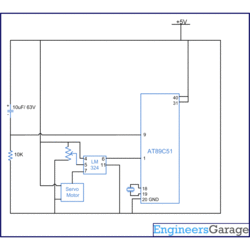# How to interface Servo Motor with 8051 Microcontroller (AT89C51)

Posted by Unknown at 9:30 PMThis project demonstrates the operation of a servo motor. The control signals for the rotation of the motor are provided by 8051 microcontroller (AT89C51). Here, the servo arm is rotated by 5° from the previous position, starting from 0 °as initial position. As the servo reaches a limit, the arm comes back to the initial position (0°). For basic concepts and know-how of a servo motor, refer to the article Servo Motor.

• The servo motor is controlled by feeding pulse width modulated (PWM) signal at the control wire of the servo motor. In addition a 4.8V (ideally 5V) DC supply is provided to the red lead of the servo. The black lead of the servo is connected to Ground.

The first pin of port P1 (P1^0) of AT89C51 microcontroller is set as the output pin to provide control signal to the servo motor. Before connecting to the control wire of servo, the output from the microcontroller is fed through a comparator IC (LM324) so that the signal is protected from any loss due to overloading.

In the program, one of the 8051 timers is configured to generate flag after every 50 µs. Usually the pulse durations corresponding to 0° and 180° angular positions are 1ms and 2ms, but these durations may vary for different servos. Here, a pulse with ON time of 700 µs sets the motor closer to 0° and a pulse of 5500 µs sets the motor to 180°. A variable is taken which is initialized to a value 14. A function using timer is written that has the variable mentioned above as an argument. This function generates time delay in the multiples of 50 µs. Initially when the microcontroller executes program, a pulse with ON time of 700 µs (14 x 50 µs) followed by OFF time of 18 ms is generated. This shifts the servo horn to 0° angle.Every time the value of variable is increased by 5. This increases the ON time of pulse by 250 µs (5 x 50 µs). So every new pulse has an ON time 250 µs greater than the previous one. This is followed by an OFF time of 18 ms. In this way, at every loop the servo is rotated by 5° ahead.
As the value of variable reaches above 100 it is again set to 14. This moves the servo back to the initial position of 0° after a full rotation.

•• ```
```
// Program to rotate servo by 5 degree from previous position starting from 0 degree

// 0 degree = 700us

// 180 degree = 5500us

// Timer1 pulse after 50us -23

#include<reg51.h>

sbit output=P1^0;    //Output to motor

int count;

void delay(unsigned int msec)   // Function for delay

{

int i,j;

for(i=0;i<msec;i++)

for(j=0;j<1275;j++);

}

void timer(int msec)  // Function for timer

{

int i;

TR1=1;

for(i=0;i<msec;i++)

{

while(TF1==0);

TF1=0;

}

TR1=0;

}

void main()

{

int i;

TMOD=0x20;   // Mode2

TH1= -23;  // 50usec timer

output=0;

count=14;

while(1)

{

if(count>=100)

count=14;

else

count=count+5;

for(i=0;i<200;i++)

{

output=1;

timer(count);

output=0;

timer(360);

}

delay(100);

}

}
```

```
• AT89C51 Microcontroller
IC LM324
Tags: